# Turán's problem for hypergraphs

 Importance: Medium ✭✭
 Author(s): Turan, Paul
 Subject: Graph Theory » Hypergraphs
 Keywords:
 Posted by: fhavet on: March 12th, 2013
Conjecture   Every simple-uniform hypergraph on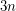vertices which contains no complete-uniform hypergraph on four vertices has at most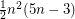hyperedges.
Conjecture   Every simple-uniform hypergraph on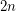vertices which contains no complete-uniform hypergraph on five vertices has at mosthyperedges.

Letbe an-set. A-uniform hypergraph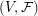is complete if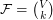, the set of all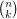-subsets of.

Letbe a partition ofinto three sets which are as nearly equal in size as possible, and letbe the union of,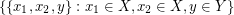,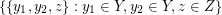, and. This-uniform hypergraph hashyperedges and contains no complete-uniform hypergraph on four vertices. Hence the first conjecture asserts that this hypergraph is extremal with this prpoerty.

Letbe a partition ofinto two sets which are as nearly equal in size as possible, and letbe the set of all-subsets ofwhich intersect bothand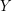. This-uniform hypergraph hashyperedges and contains no complete-uniform hypergraph on five vertices. Hence the second conjecture asserts that this hypergraph is extremal with this property.

## Bibliography

*[T] P. Turán, Eine Extremalaufgabe aus der Graphentheorie. Mat. Fiz. Lapok 48 (1941), 436--452.

* indicates original appearance(s) of problem.